Скачать презентацию EKT 104 ANALOG ELECTRONIC CIRCUITS LITAR ELEKTRONIK ANALOG

3fcf3540065b93cf0d5be6c2df391c77.ppt

• Количество слайдов: 29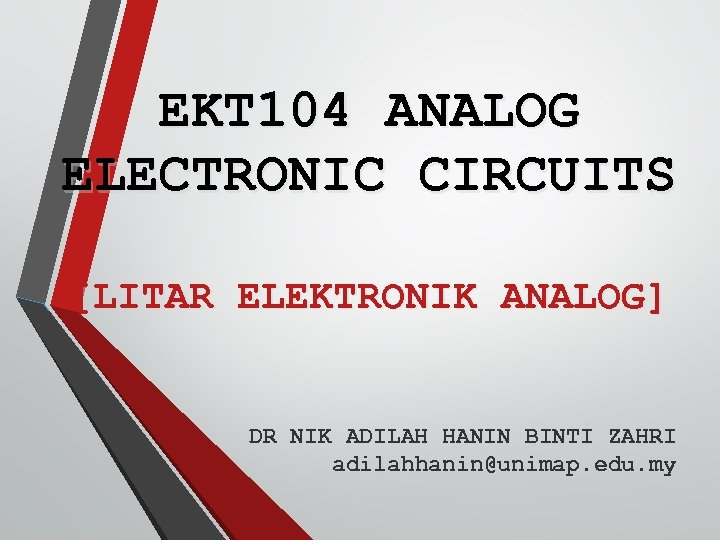EKT 104 ANALOG ELECTRONIC CIRCUITS [LITAR ELEKTRONIK ANALOG] DR NIK ADILAH HANIN BINTI ZAHRI [email protected] edu. my 1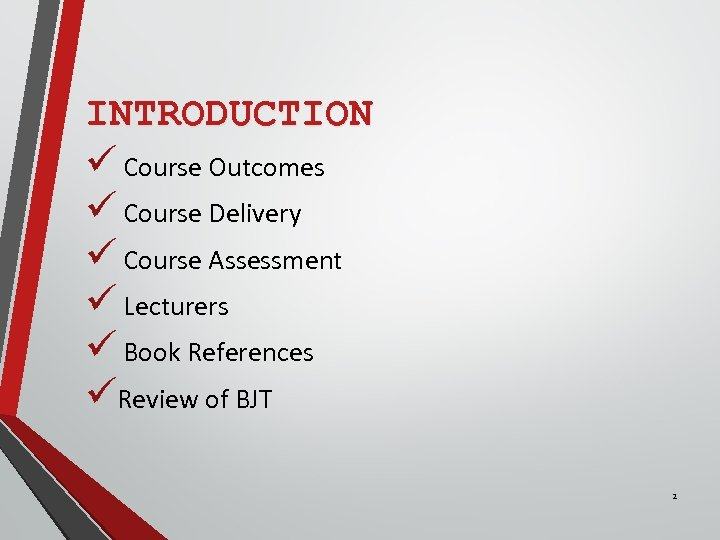INTRODUCTION ü Course Outcomes ü Course Delivery ü Course Assessment ü Lecturers ü Book References üReview of BJT 2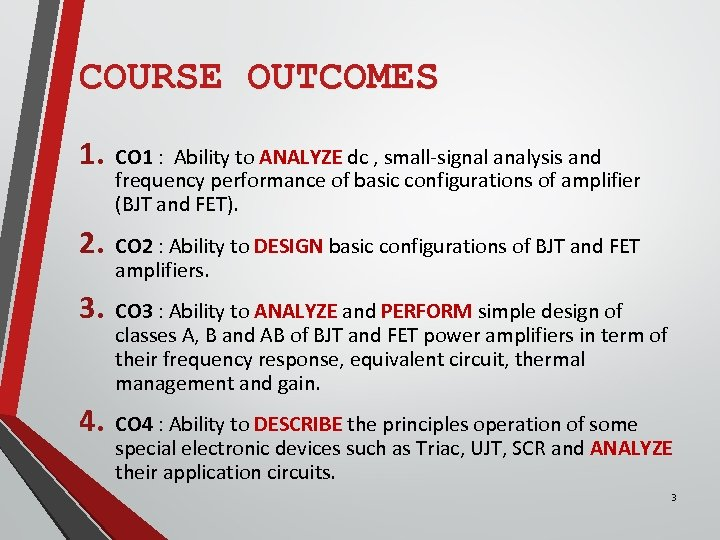COURSE OUTCOMES 1. CO 1 : Ability to ANALYZE dc , small-signal analysis and frequency performance of basic configurations of amplifier (BJT and FET). 2. CO 2 : Ability to DESIGN basic configurations of BJT and FET amplifiers. 3. CO 3 : Ability to ANALYZE and PERFORM simple design of classes A, B and AB of BJT and FET power amplifiers in term of their frequency response, equivalent circuit, thermal management and gain. 4. CO 4 : Ability to DESCRIBE the principles operation of some special electronic devices such as Triac, UJT, SCR and ANALYZE their application circuits. 3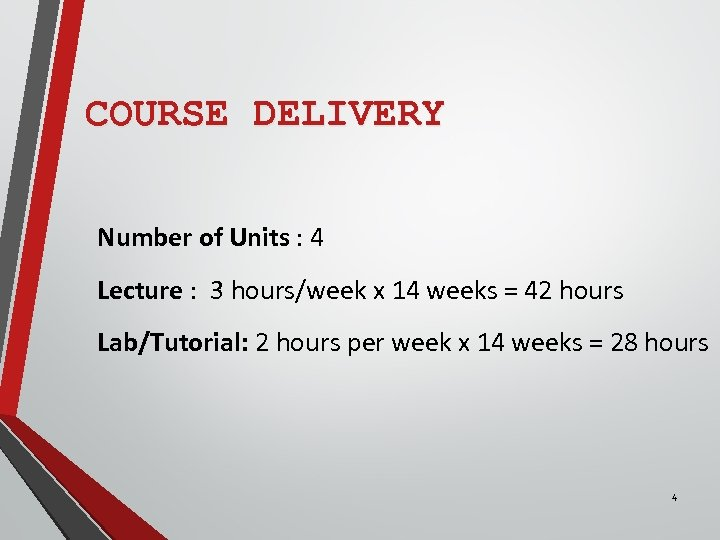COURSE DELIVERY Number of Units : 4 Lecture : 3 hours/week x 14 weeks = 42 hours Lab/Tutorial: 2 hours per week x 14 weeks = 28 hours 4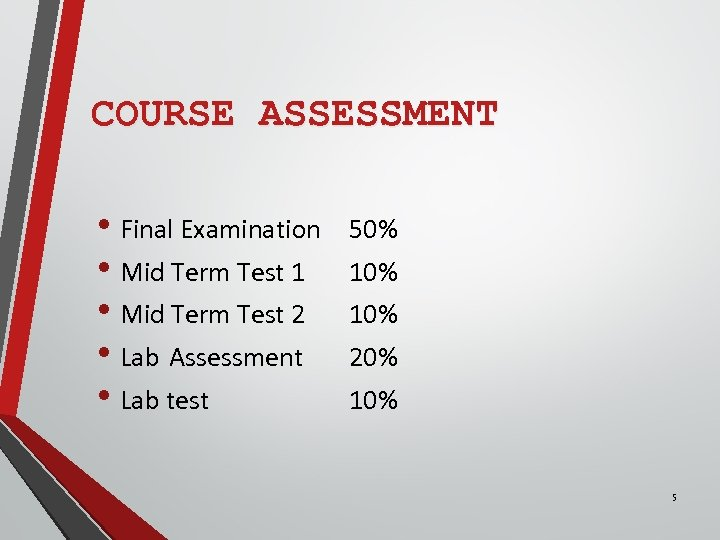COURSE ASSESSMENT • Final Examination • Mid Term Test 1 • Mid Term Test 2 • Lab Assessment • Lab test 50% 10% 20% 10% 5LECTURERS • DR NIK ADILAH HANIN BT ZAHRI [email protected] edu. my • DR ONG BI LYNN [email protected] edu. my • DR AMIZA AMIR [email protected] com 6BOOK REFERENCES Textbook: • Donald A. Neamen, ‘MICROELECTRONICS Circuit Analysis & Design’, 3 rd Edition’, Mc. Graw Hill International Edition, 2007 Reference Books: • Boylestad, R. L. , Nashelsky, L. , “Electronic Devices and Circuit Theory”, 8 th Edition, Prentice Hall, 2002. • Thomas L. Floyd, ‘Electronic devices: Conventional Current Version’, 7 th ed. ’, Prentice Hall • Gates, E. D. , “Introduction to Electronics”, 5 th Edition, Delmar Cengage Learning. 7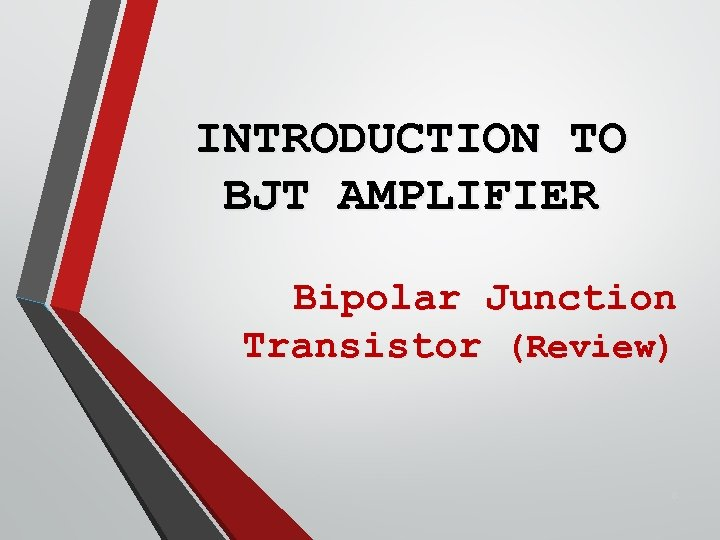INTRODUCTION TO BJT AMPLIFIER Bipolar Junction Transistor (Review) 8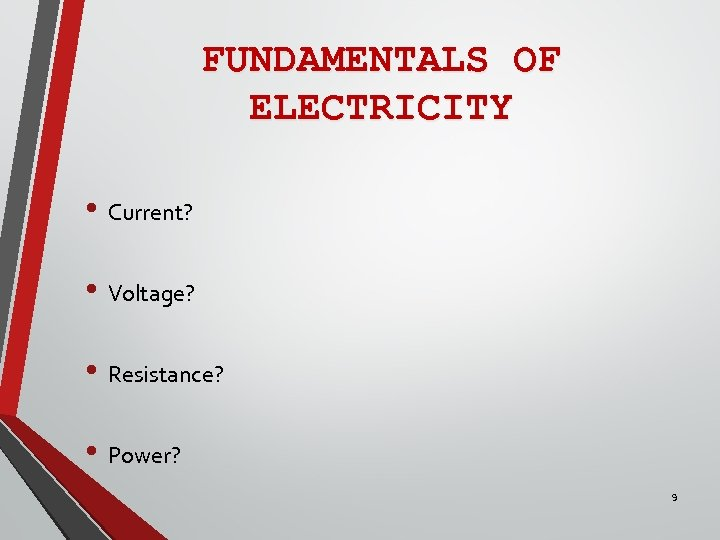FUNDAMENTALS OF ELECTRICITY • Current? • Voltage? • Resistance? • Power? 9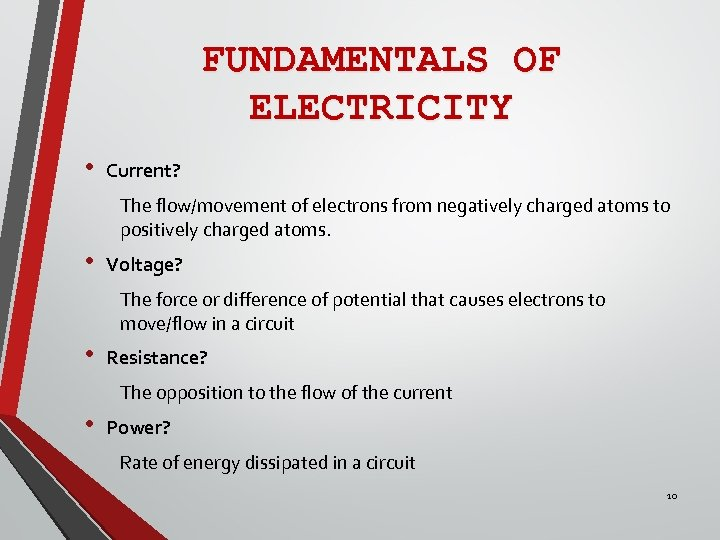FUNDAMENTALS OF ELECTRICITY • Current? The flow/movement of electrons from negatively charged atoms to positively charged atoms. • Voltage? The force or difference of potential that causes electrons to move/flow in a circuit • Resistance? The opposition to the flow of the current • Power? Rate of energy dissipated in a circuit 10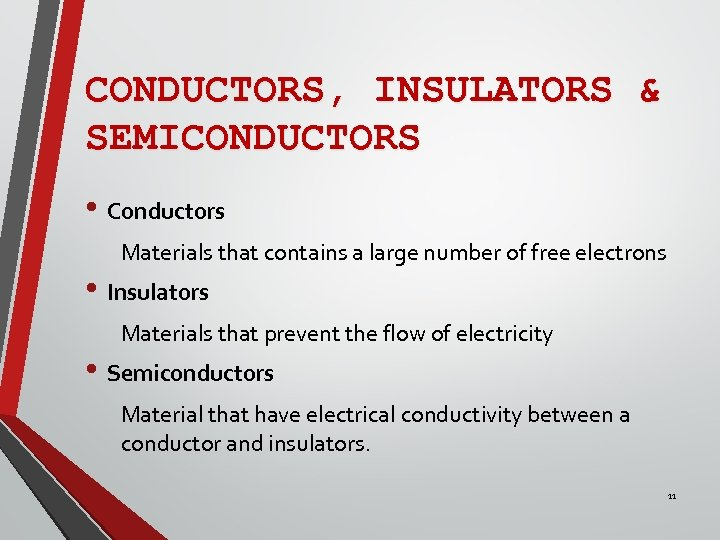CONDUCTORS, INSULATORS & SEMICONDUCTORS • Conductors Materials that contains a large number of free electrons • Insulators Materials that prevent the flow of electricity • Semiconductors Material that have electrical conductivity between a conductor and insulators. 11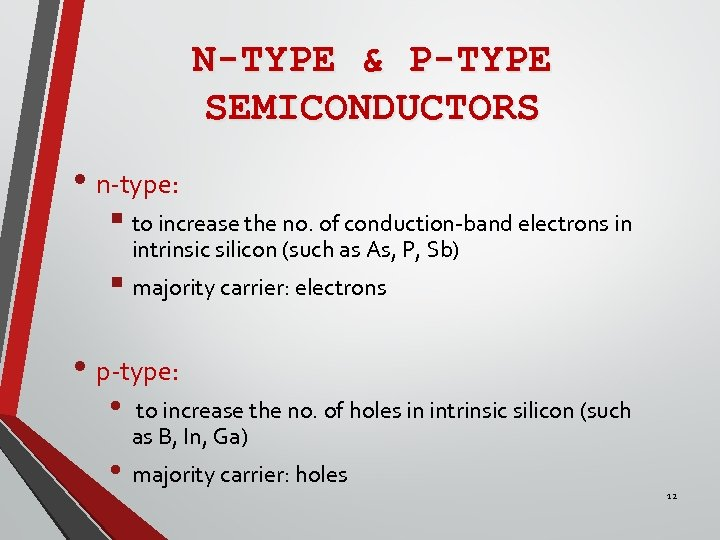N-TYPE & P-TYPE SEMICONDUCTORS • n-type: § to increase the no. of conduction-band electrons in intrinsic silicon (such as As, P, Sb) § majority carrier: electrons • p-type: • to increase the no. of holes in intrinsic silicon (such as B, In, Ga) • majority carrier: holes 12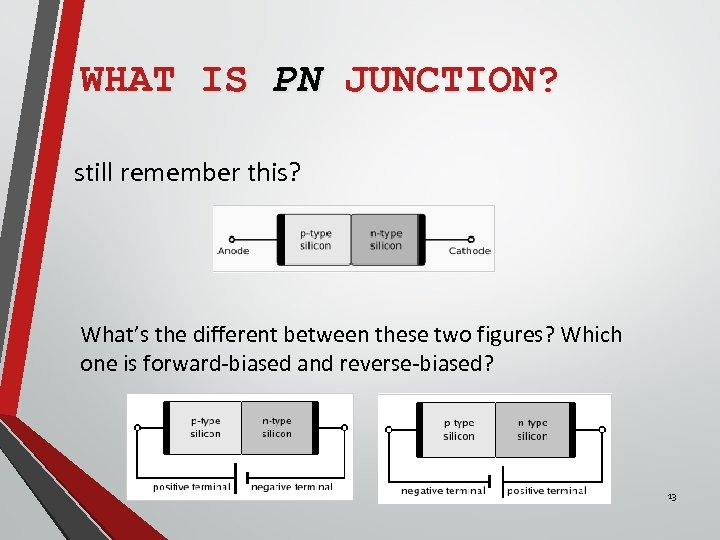WHAT IS PN JUNCTION? still remember this? What’s the different between these two figures? Which one is forward-biased and reverse-biased? 13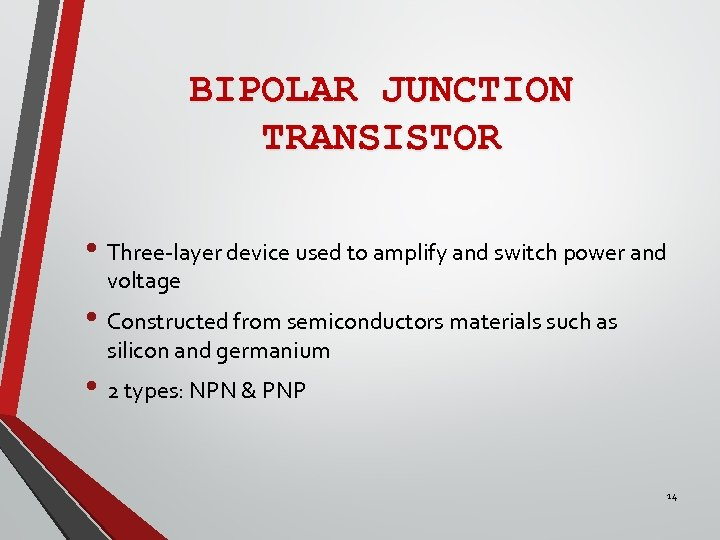BIPOLAR JUNCTION TRANSISTOR • Three-layer device used to amplify and switch power and voltage • Constructed from semiconductors materials such as silicon and germanium • 2 types: NPN & PNP 14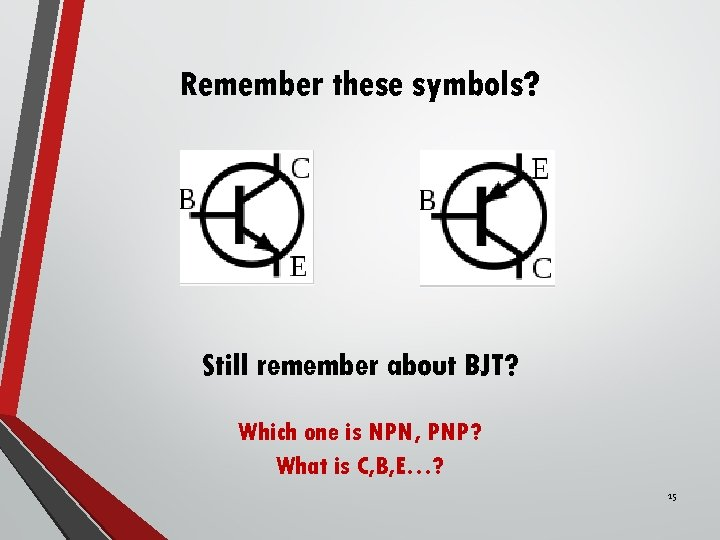Remember these symbols? Still remember about BJT? Which one is NPN, PNP? What is C, B, E…? 15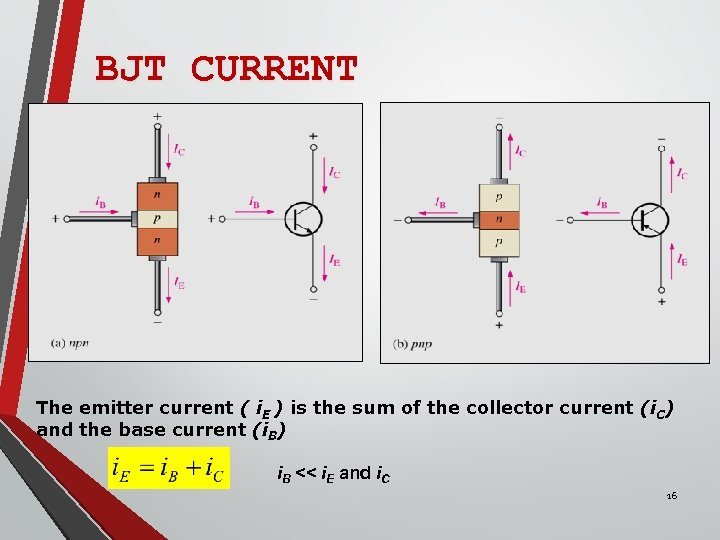BJT CURRENT The emitter current ( i. E ) is the sum of the collector current (i. C) and the base current (i. B) i. B << i. E and i. C 16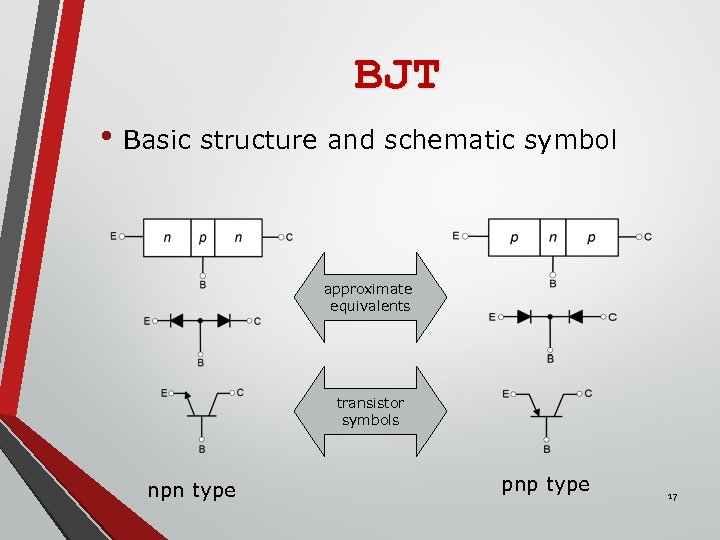BJT • Basic structure and schematic symbol approximate equivalents transistor symbols npn type pnp type 17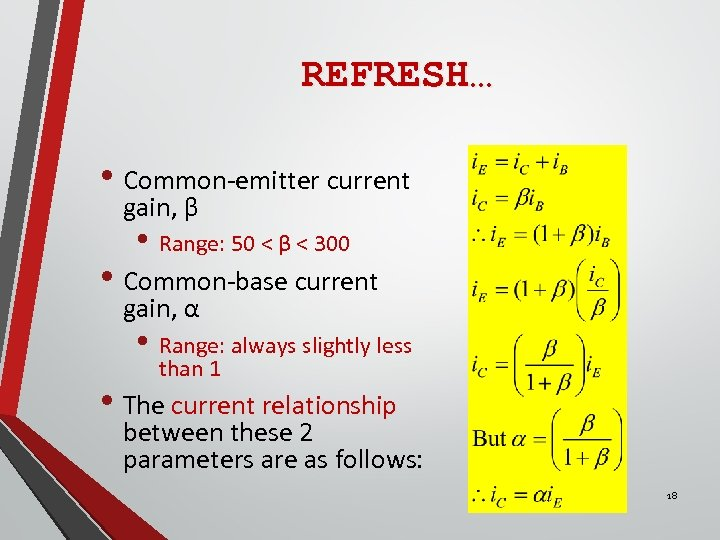REFRESH… • Common-emitter current gain, β • Range: 50 < β < 300 • Common-base current gain, α • Range: always slightly less than 1 • The current relationship between these 2 parameters are as follows: 18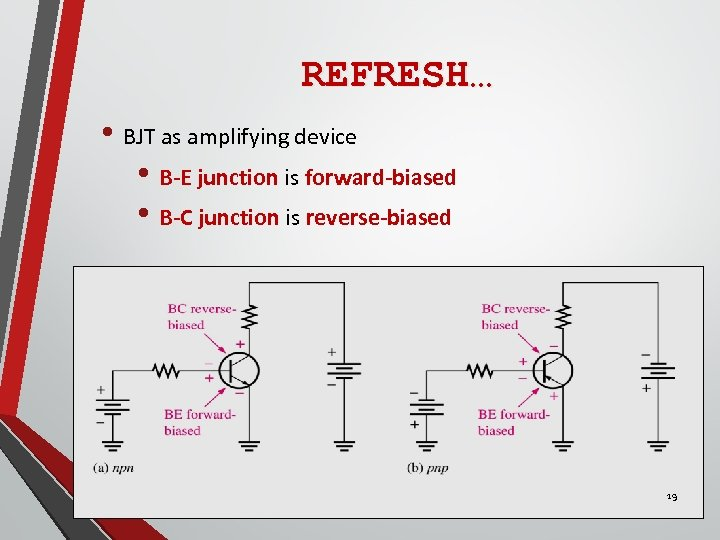REFRESH… • BJT as amplifying device • B-E junction is forward-biased • B-C junction is reverse-biased 19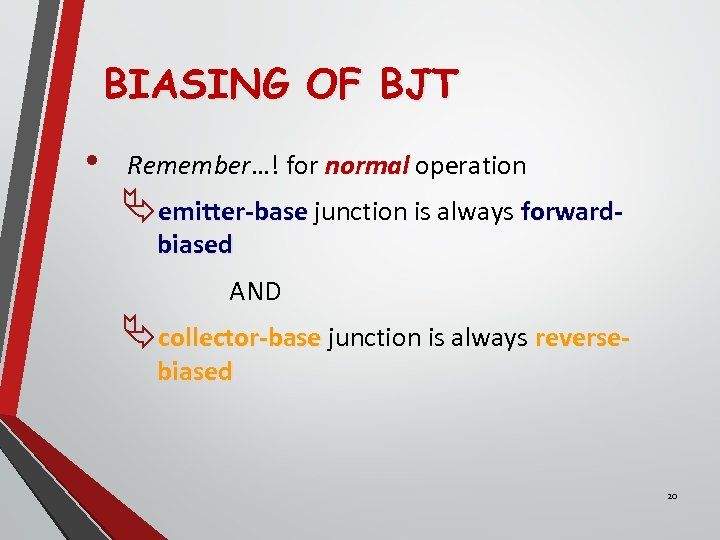BIASING OF BJT • Remember…! for normal operation Äemitter-base junction is always forwardbiased AND Äcollector-base junction is always reversebiased 20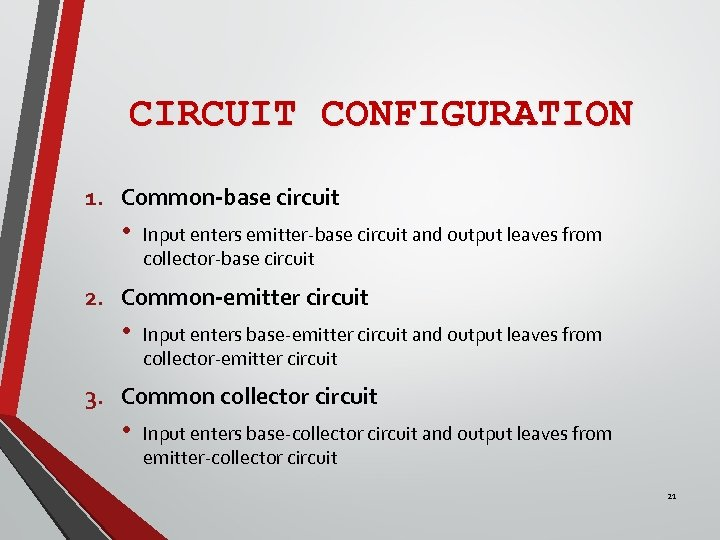CIRCUIT CONFIGURATION 1. Common-base circuit • Input enters emitter-base circuit and output leaves from collector-base circuit 2. Common-emitter circuit • Input enters base-emitter circuit and output leaves from collector-emitter circuit 3. Common collector circuit • Input enters base-collector circuit and output leaves from emitter-collector circuit 21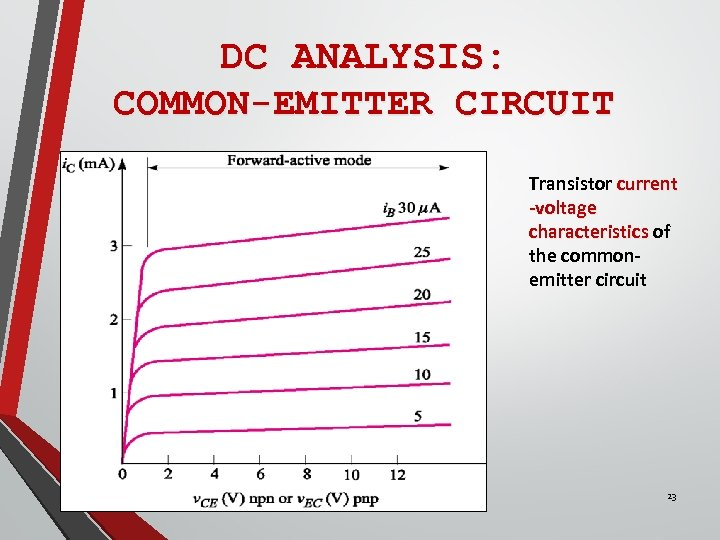DC ANALYSIS: COMMON-EMITTER CIRCUIT Transistor current -voltage characteristics of the commonemitter circuit 23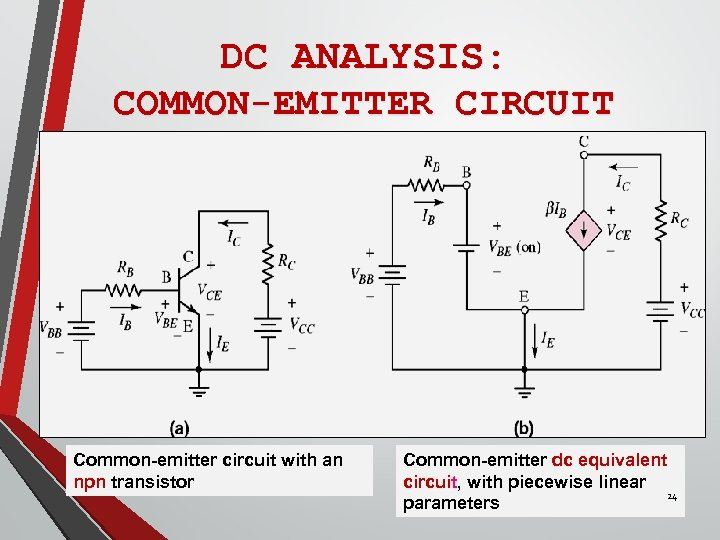DC ANALYSIS: COMMON-EMITTER CIRCUIT Common-emitter circuit with an npn transistor Common-emitter dc equivalent circuit, with piecewise linear 24 parameters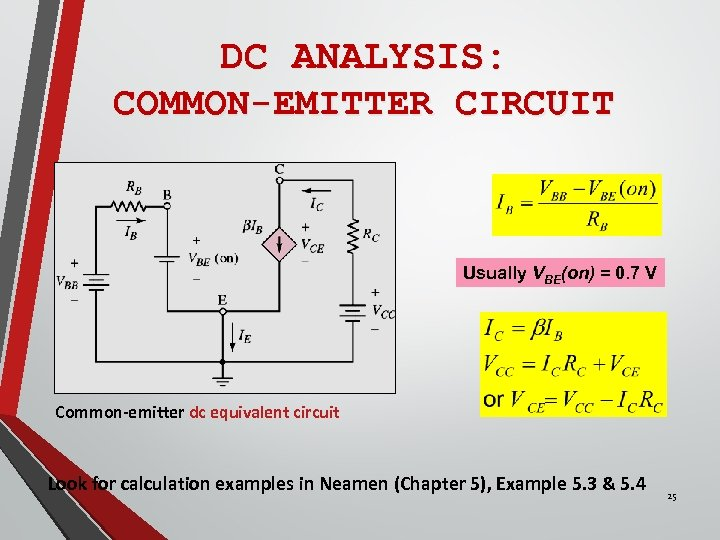DC ANALYSIS: COMMON-EMITTER CIRCUIT Usually VBE(on) = 0. 7 V Common-emitter dc equivalent circuit Look for calculation examples in Neamen (Chapter 5), Example 5. 3 & 5. 4 25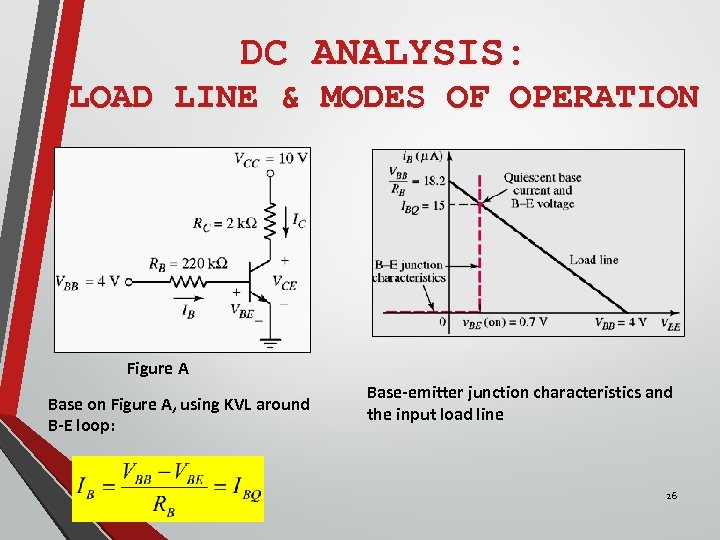DC ANALYSIS: LOAD LINE & MODES OF OPERATION Figure A Base on Figure A, using KVL around B-E loop: Base-emitter junction characteristics and the input load line 26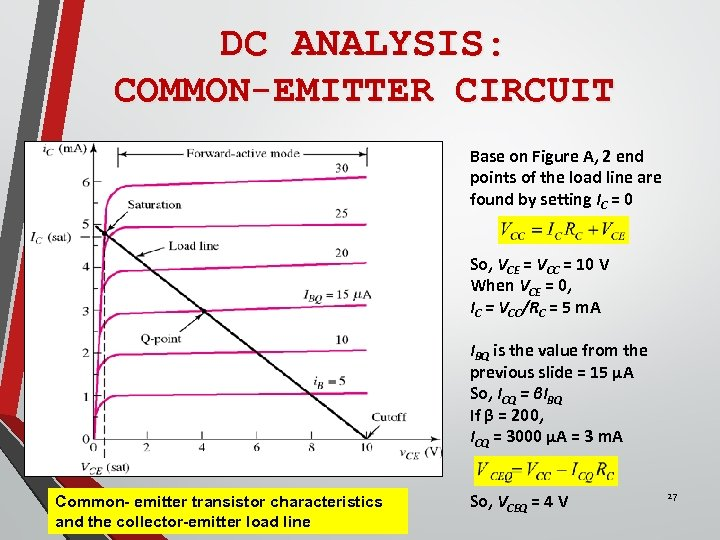DC ANALYSIS: COMMON-EMITTER CIRCUIT Base on Figure A, 2 end points of the load line are found by setting IC = 0 So, VCE = VCC = 10 V When VCE = 0, IC = VCC/RC = 5 m. A IBQ is the value from the previous slide = 15 µA So, ICQ = βIBQ If β = 200, ICQ = 3000 µA = 3 m. A Common- emitter transistor characteristics and the collector-emitter load line So, VCEQ = 4 V 27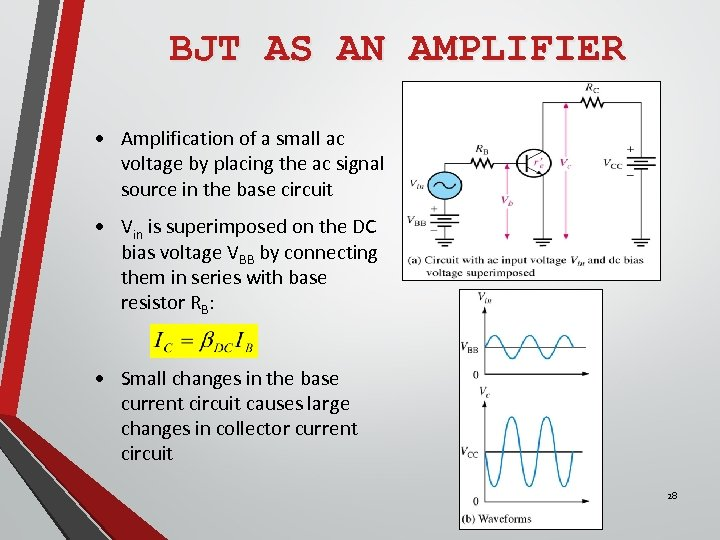BJT AS AN AMPLIFIER • Amplification of a small ac voltage by placing the ac signal source in the base circuit • Vin is superimposed on the DC bias voltage VBB by connecting them in series with base resistor RB: • Small changes in the base current circuit causes large changes in collector current circuit 28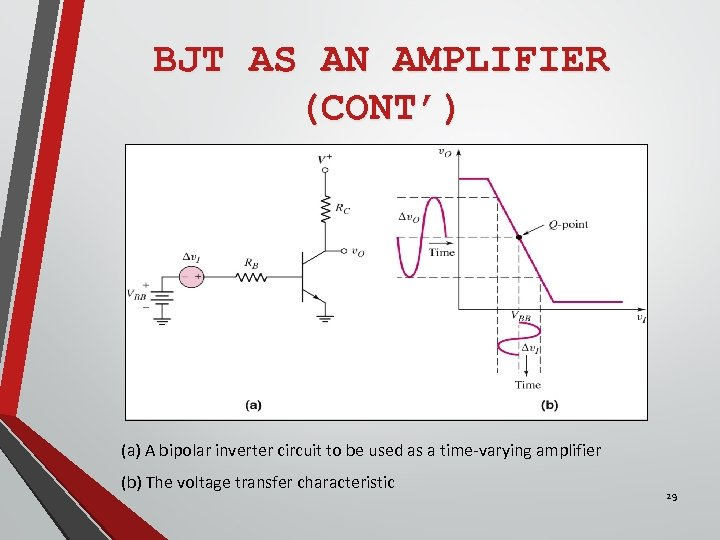BJT AS AN AMPLIFIER (CONT’) (a) A bipolar inverter circuit to be used as a time-varying amplifier (b) The voltage transfer characteristic 29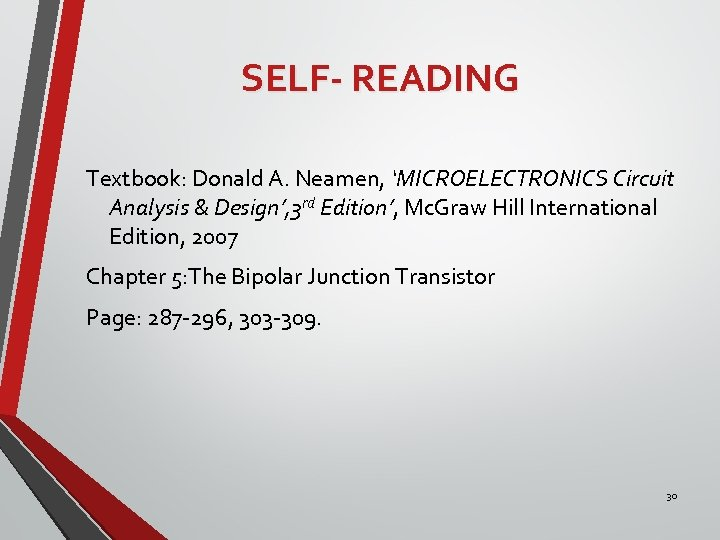SELF- READING Textbook: Donald A. Neamen, ‘MICROELECTRONICS Circuit Analysis & Design’, 3 rd Edition’, Mc. Graw Hill International Edition, 2007 Chapter 5: The Bipolar Junction Transistor Page: 287 -296, 303 -309. 30